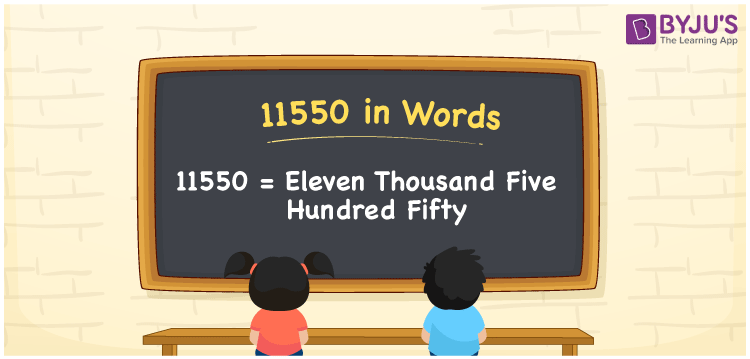# 11550 in Words

11550 in words is written as Eleven thousand five hundred fifty. In both the International System of Numerals and the Indian System of Numerals, 11550 is written as Eleven thousand five hundred fifty. The number 11550 is a Cardinal Number as it represents some quantity. For example, “that mobile phone costs 11550 rupees”.

 11550 in Words Eleven thousand five hundred fifty Eleven thousand five hundred fifty in numerical form 11550

## 11550 in English Words

11550 in English words is read as “Eleven thousand five hundred fifty”## How to Write 11550 in Words?

To write 11550 in words, we shall use the place value chart. In the place value chart, put 1 in the ten thousands and thousands, 5 in the hundreds and tens and 0 in the ones, respectively. Let us make a place value chart to write the number 11550 in words.

 Ten Thousands Thousands Hundreds Tens Ones 1 1 5 5 0

Thus, we can write the expanded form as

1 × Ten Thousand + 1 × Thousand + 5 × Hundred + 5 × Ten + 0 × One

= 1 × 10000 + 1 × 1000 + 5 × 100 + 5 × 10 + 0 × 1

= 10000 + 1000 + 500 + 50 + 0

= 11550

= Eleven thousand five hundred fifty.

11550 is a natural number, the successor of 11549 and the predecessor of 11551.

11550 in words – Eleven thousand five hundred fifty

• Is 11550 an odd number? – No
• Is 11550 an even number? – Yes
• Is 11550 a perfect square number? – No
• Is 11550 a perfect cube number? – No
• Is 11550 a prime number? – No
• Is 11550 a composite number? – Yes

## Frequently Asked Questions on 11550 in Words

Q1

### How to write 11550 in words?

11550 in words is written as Eleven thousand five hundred fifty.
Q2

### How to write 11550 in the International and Indian System of Numerals?

In both, the system of numerals, 11550 in words, is written as Eleven thousand five hundred fifty.
Q3

### How to write 11550 in a place value chart?

In the place value chart, write 1 in the ten thousands and thousands, 5 in the hundreds and tens and 0 in the ones, respectively.
Test your Knowledge on 11550 in Words